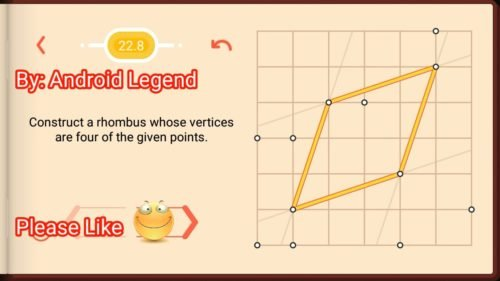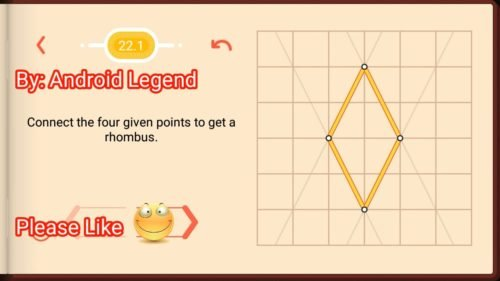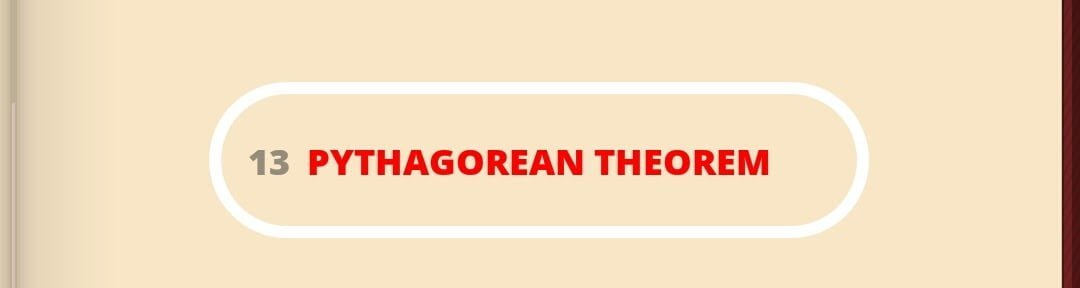# Pythagorea Rhombuses Level 22.8 Solution/AnswerPythagorea Rhombuses Level 22.8 New Version Game Answers,  detailed solutions, Tips, and Walkthrough. Scroll below to find answer to this level.

Pythagorea is android/iOS app developed by Horis International Limited. Solutions hints and answers to pythagorea are available in this post scroll down to find solutions to all the levels.

This game is mostly focused on geometric puzzles and construction. The work space is divided into grids to draw lines. You should know all the basic Math operations. All lines and shapes are drawn on a grid whose cells are squares. Most of the game levels can be answered using natural intuition and by some basic laws of geometry.

Click Here for All other Pythagorea Levels:• Pythagorea Level 22.8: Construct a rhombus whose Vertices
are four of the given points.If you have any doubt regarding Pythagorea answers given here you can watch video below or you can comment on this post-

# Pythagorea Rhombuses Level 22.7 Solution/AnswerPythagorea Rhombuses Level 22.7 New Version Game Answers,  detailed solutions, Tips, and Walkthrough. Scroll below to find answer to this level.

Pythagorea is android/iOS app developed by Horis International Limited. Solutions hints and answers to pythagorea are available in this post scroll down to find solutions to all the levels.

This game is mostly focused on geometric puzzles and construction. The work space is divided into grids to draw lines. You should know all the basic Math operations. All lines and shapes are drawn on a grid whose cells are squares. Most of the game levels can be answered using natural intuition and by some basic laws of geometry.

Click Here for All other Pythagorea Levels:• Pythagorea Level 22.7: Construct a rhombus whose Vertices are four of the given points.If you have any doubt regarding Pythagorea answers given here you can watch video below or you can comment on this post-

# Pythagorea Rhombuses Level 22.6 Solution/AnswerPythagorea Rhombuses Level 22.6 New Version Game Answers,  detailed solutions, Tips, and Walkthrough. Scroll below to find answer to this level.

Pythagorea is android/iOS app developed by Horis International Limited. Solutions hints and answers to pythagorea are available in this post scroll down to find solutions to all the levels.

This game is mostly focused on geometric puzzles and construction. The work space is divided into grids to draw lines. You should know all the basic Math operations. All lines and shapes are drawn on a grid whose cells are squares. Most of the game levels can be answered using natural intuition and by some basic laws of geometry.

Click Here for All other Pythagorea Levels:• Pythagorea Level 22.6: Inscribe a rhombus in a given parallelogram so that they share a diagonal. One of its vertices is in the point A.If you have any doubt regarding Pythagorea answers given here you can watch video below or you can comment on this post-

# Pythagorea Rhombuses Level 22.5 Solution/AnswerPythagorea Rhombuses Level 22.5 New Version Game Answers,  detailed solutions, Tips, and Walkthrough. Scroll below to find answer to this level.

Pythagorea is android/iOS app developed by Horis International Limited. Solutions hints and answers to pythagorea are available in this post scroll down to find solutions to all the levels.

This game is mostly focused on geometric puzzles and construction. The work space is divided into grids to draw lines. You should know all the basic Math operations. All lines and shapes are drawn on a grid whose cells are squares. Most of the game levels can be answered using natural intuition and by some basic laws of geometry.

Click Here for All other Pythagorea Levels:• Pythagorea Level 22.5: Construct all rhombuses whose vertices are nodes (one of them is given) with one diagonal of length 4 and the other of length 6.If you have any doubt regarding Pythagorea answers given here you can watch video below or you can comment on this post-

# Pythagorea Rhombuses Level 22.4 Solution/AnswerPythagorea Rhombuses Level 22.4 New Version Game Answers,  detailed solutions, Tips, and Walkthrough. Scroll below to find answer to this level.

Pythagorea is android/iOS app developed by Horis International Limited. Solutions hints and answers to pythagorea are available in this post scroll down to find solutions to all the levels.

This game is mostly focused on geometric puzzles and construction. The work space is divided into grids to draw lines. You should know all the basic Math operations. All lines and shapes are drawn on a grid whose cells are squares. Most of the game levels can be answered using natural intuition and by some basic laws of geometry.

Click Here for All other Pythagorea Levels:• Pythagorea Level 22.4: Construct all rhombuses whose vertices are nodes given two of them.If you have any doubt regarding Pythagorea answers given here you can watch video below or you can comment on this post-

# Pythagorea Rhombuses Level 22.3 Solution/AnswerPythagorea Rhombuses Level 22.3 New Version Game Answers,  detailed solutions, Tips, and Walkthrough. Scroll below to find answer to this level.

Pythagorea is android/iOS app developed by Horis International Limited. Solutions hints and answers to pythagorea are available in this post scroll down to find solutions to all the levels.

This game is mostly focused on geometric puzzles and construction. The work space is divided into grids to draw lines. You should know all the basic Math operations. All lines and shapes are drawn on a grid whose cells are squares. Most of the game levels can be answered using natural intuition and by some basic laws of geometry.

Click Here for All other Pythagorea Levels:• Pythagorea Level 22.3: Construct a rhombus given three of its Vertices.If you have any doubt regarding Pythagorea answers given here you can watch video below or you can comment on this post-

# Pythagorea Rhombuses Level 22.2 Solution/AnswerPythagorea Rhombuses Level 22.2 New Version Game Answers,  detailed solutions, Tips, and Walkthrough. Scroll below to find answer to this level.

Pythagorea is android/iOS app developed by Horis International Limited. Solutions hints and answers to pythagorea are available in this post scroll down to find solutions to all the levels.

This game is mostly focused on geometric puzzles and construction. The work space is divided into grids to draw lines. You should know all the basic Math operations. All lines and shapes are drawn on a grid whose cells are squares. Most of the game levels can be answered using natural intuition and by some basic laws of geometry.

Click Here for All other Pythagorea Levels:• Pythagorea Level 22.2: Construct a rhombus given three of its Vertices.If you have any doubt regarding Pythagorea answers given here you can watch video below or you can comment on this post-

# Pythagorea Rhombuses Level 22.1 Solution/AnswerPythagorea Rhombuses Level 22.1 New Version Game Answers,  detailed solutions, Tips, and Walkthrough. Scroll below to find answer to this level.

Pythagorea is android/iOS app developed by Horis International Limited. Solutions hints and answers to pythagorea are available in this post scroll down to find solutions to all the levels.

This game is mostly focused on geometric puzzles and construction. The work space is divided into grids to draw lines. You should know all the basic Math operations. All lines and shapes are drawn on a grid whose cells are squares. Most of the game levels can be answered using natural intuition and by some basic laws of geometry.

Click Here for All other Pythagorea Levels:• Pythagorea Level 22.1: Connect the four given points to get a rhombus.If you have any doubt regarding Pythagorea answers given here you can watch video below or you can comment on this post-

# Pythagorea Pythagorean Theorem Level 13.15 Solution/AnswerPythagorea Pythagorean Theorem Level 13.15 New Version Game Answers,  detailed solutions, Tips, and Walkthrough. Scroll below to find answer to this level.

Pythagorea is android/iOS app developed by Horis International Limited. Solutions hints and answers to pythagorea are available in this post scroll down to find solutions to all the levels.

This game is mostly focused on geometric puzzles and construction. The work space is divided into grids to draw lines. You should know all the basic Math operations. All lines and shapes are drawn on a grid whose cells are squares. Most of the game levels can be answered using natural intuition and by some basic laws of geometry.

Click Here for All other Pythagorea Levels:Pythagorea Level 13.15: Construct all isosceles triangles Whose Vertices are nodes and Whose leg length is root 61.If you have any doubt regarding Pythagorea answers given here you can watch video below or you can comment on this post-

# Pythagorea Pythagorean Theorem Level 13.14 Solution/AnswerPythagorea Pythagorean Theorem Level 13.14 New Version Game Answers,  detailed solutions, Tips, and Walkthrough. Scroll below to find answer to this level.

Pythagorea is android/iOS app developed by Horis International Limited. Solutions hints and answers to pythagorea are available in this post scroll down to find solutions to all the levels.

This game is mostly focused on geometric puzzles and construction. The work space is divided into grids to draw lines. You should know all the basic Math operations. All lines and shapes are drawn on a grid whose cells are squares. Most of the game levels can be answered using natural intuition and by some basic laws of geometry.

Click Here for All other Pythagorea Levels:Pythagorea Level 13.14: Construct all rhombuses whose vertices are nodes with one diagonal of length root 32 and the other of length root 72.If you have any doubt regarding Pythagorea answers given here you can watch video below or you can comment on this post-